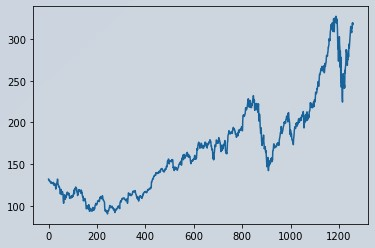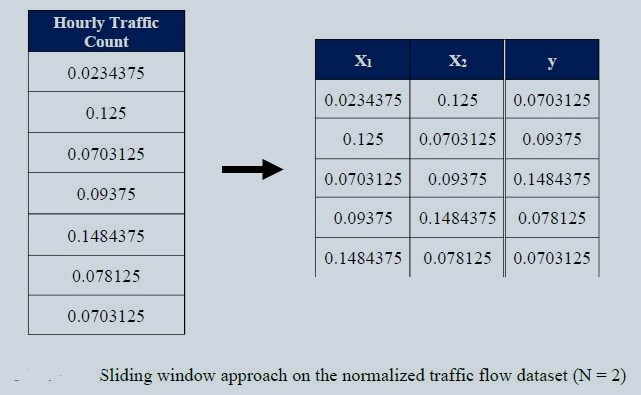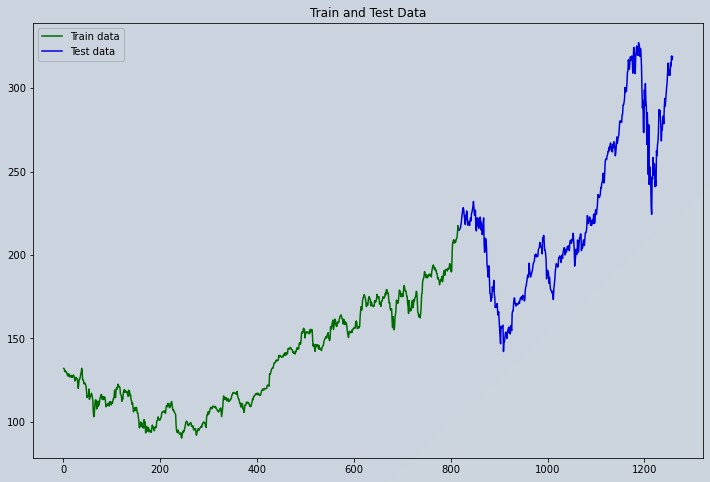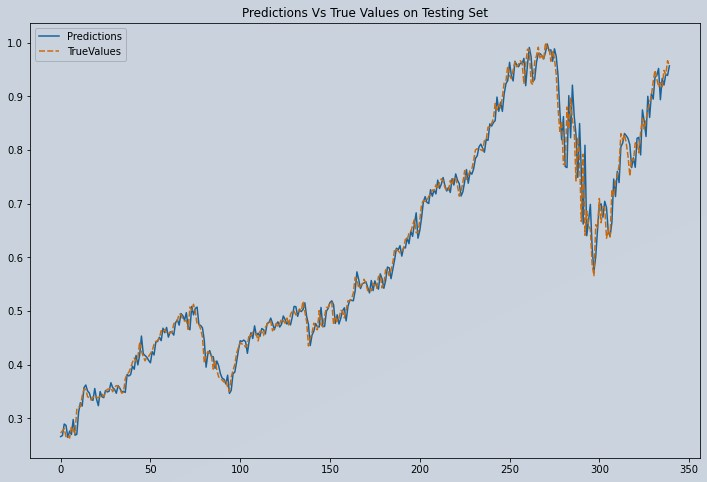## Introduction

Stock market price prediction sounds fascinating but is equally difficult. In this article, we will show you how to write a python program that predicts the price of stock using machine learning algorithm called Linear Regression. We will work with historical data of APPLE company. The data shows the stock price of APPLE from 2015-05-27 to 2020-05-22. In this article, our aim is to implement a machine learning algorithm (Linear Regression) to predict stock price of APPLE company.

Table of Content

Let’s see how to predict stock prices using Machine Learning and the python programming language. we will start this task by importing all the necessary python libraries that we need for this task:

```    # Importing libraries
import numpy as np
from numpy import array
import pandas as pd
from sklearn import preprocessing
from sklearn.model_selection import train_test_split
from sklearn.linear_model import LinearRegression
import matplotlib.pyplot as plt
import seaborn as sns
from sklearn.preprocessing import MinMaxScaler
from sklearn.linear_model import LinearRegression
import math
from sklearn.metrics import mean_squared_error

```

## Data Preprocessing

We would be using the Apple Inc. stock scrip data for this project. We have a historic data set from 27th May 2015 to 22nd May 2020. A copy of the data used is kept over here. Click on the Apple Stock Download data to get a csv file format copied on your disk.

```    df = pd.read_csv('AAPL.csv')

```

We will have a look at the dataset using df.head(), it will show the first 5 entries of the dataset.

```    pd.set_option('display.max_columns', None)

0           0   AAPL  2015-05-27 00:00:00+00:00  132.045  132.260  130.05  130.34  45833246  121.682558  121.880685  119.844118  120.111360  45833246      0.0          1.0
1           1   AAPL  2015-05-28 00:00:00+00:00  131.780  131.950  131.10  131.86  30733309  121.438354  121.595013  120.811718  121.512076  30733309      0.0          1.0
2           2   AAPL  2015-05-29 00:00:00+00:00  130.280  131.450  129.90  131.23  50884452  120.056069  121.134251  119.705890  120.931516  50884452      0.0          1.0
3           3   AAPL  2015-06-01 00:00:00+00:00  130.535  131.390  130.05  131.20  32112797  120.291057  121.078960  119.844118  120.903870  32112797      0.0          1.0
4           4   AAPL  2015-06-02 00:00:00+00:00  129.960  130.655  129.32  129.86  33667627  119.761181  120.401640  119.171406  119.669029  33667627      0.0          1.0

[5 rows x 15 columns]

# Closing Price
df1 = df['close']
df['close'].plot()

```

###Figure 1 : Apple Stock Market Data Visualization

### Scaling Data

Before we begin our model fitting, lets normalize this data. This will boost the performance. It is clear that the df1 is a vector. But the problem is MinMaxScaler works on numpy 2D arrays, not on vectors. So, we will convert df1 to 2D array using np.array(df1).reshape(-1,1)) and then apply the scaling.

```    df1 = np.array(df1)
df1 = df1.reshape(-1,1)

scaler = MinMaxScaler(feature_range=(0,1))
df1 = scaler.fit_transform(df1)
print(df1)

[[0.17607447]
[0.17495567]
[0.16862282]
...
[0.96635143]
[0.9563033 ]
[0.96491598]]

```

## Splitting Data into Training and Testing Set

```    df1.shape
(1258, 1)

```

In this analysis we will split the dataset into 65% training and 35% testing set. Lets split our data into training and testing sets as a standard process.

```    # splitting dataset into train and test split
training_size = int(len(df1)*0.65)
test_size = len(df1)-training_size
train_data,test_data  =df1[0:training_size,:], df1[training_size:len(df1),:1]
train_data.shape
(817, 1)

test_data.shape
(441, 1)

training_size, test_size
(817, 441)

train_data[:10]
array([[0.17607447],
[0.17495567],
[0.16862282],
[0.1696994 ],
[0.16727181],
[0.16794731],
[0.16473866],
[0.16174111],
[0.1581525 ],
[0.15654817]])

```

### Converting Array of Matrix into a Dataset Matrix

Now we will write a function that will prepare the dataset so that we can fit it easily in the Linear Regression model.

### Windowing Dataset

For better performance of any time series (univariate), it is necessary to use the splitting window on the dataset. The concept is simple. We will convert the dataset into several overlapping series. You will have an idea by seeing the picture below.Figure 2 : Specimen Sliding Window Approach on Normalized Traffic Flow Data

Figure 2, shows the window size = 2. We will be using suitable window size for the best performance. You can try with any number you want. It is a hyper parameter that is needed to be tuned.

```    def create_dataset(dataset, time_step=1):
dataX, dataY = [], []
for i in range(len(dataset)-time_step-1):
a= dataset[i:(i+time_step), 0]
dataX.append(a)
dataY.append(dataset[i+ time_step, 0])
return np.array(dataX), np.array(dataY)

```

Let's choose window size = 100 for now and apply the windowing on training and testing data's

```    time_step = 100
X_train, y_train = create_dataset(train_data, time_step)
X_test, y_test = create_dataset(test_data, time_step)
train_data.shape, test_data.shape
((817, 1), (441, 1))

```
```    # A total of 817 + 441 = 1258
# allocate series of 817  from index 1 to 817
trainplot = np.arange(1,818)
# allocate series of 818 to 1258
testplot = np.arange(818,1259)

```
```    # Ploting Train and Test Data
plt.figure(figsize=(12,8))
plt.plot(trainplot,scaler.inverse_transform(train_data)[:,0], 'green', label='Train data')
plt.plot(testplot, scaler.inverse_transform(test_data)[:,0],'blue', label='Test data')
plt.legend()
plt.title('Train and Test Data')
plt.show()

```

##Figure 3 : Apple Stock Market Data Visualization Train and Test Series

## Model Building (linear Regression)

Now it's time to build our model ::::: LinearRegression

```    model = LinearRegression()
model.fit(X_train, y_train)

LinearRegression()

```

## Predictions and Model Evaluation

Predictions of Testing Set ::::: Now we visualize how our models perform within the test set

```    predictions = model.predict(X_test)
print("Predicted Value",predictions[:10])
print("Expected Value",y_test[:10])

Predicted Value 0.26591241262096627
Expected Value 0.2727349489149709

pred_df= pd.DataFrame(predictions)
pred_df['TrueValues']=y_test

new_pred_df=pred_df.rename(columns={0: 'Predictions'})

Predictions  TrueValues
0     0.265912    0.272735
1     0.267869    0.276619
2     0.289373    0.280672
3     0.286837    0.265811
4     0.264365    0.268429
```

## Plot Predicted vs Actual Prices of Test Series

```    plt.figure(figsize=(12,8))
sns.lineplot(data=new_pred_df)
plt.title("Predictions Vs True Values on Testing Set")

Text(0.5, 1.0, 'Predictions Vs True Values on Testing Set')
```Figure 4: Plot of Predicted vs Actual Apple Stock Test Data

```    print("model Accuracy on training data:",model.score(X_train, y_train))

model Accuracy on training data: 0.9970342320018716

# Model accuracy on Testing data
print("model Accuracy is on training data:",model.score(X_test, y_test))

model Accuracy on testing data: 0.9847722212152704

# Lets Do the prediction and check performance metrics
train_predict = model.predict(X_train)
test_predict = model.predict(X_test)
train_predict = train_predict.reshape(-1, 1)
test_predict = test_predict.reshape(-1, 1)

# Transform back to original form
train_predict = scaler.inverse_transform(train_predict)
test_predict = scaler.inverse_transform(test_predict)

# Calculate RMSE performance metrics
math.sqrt(mean_squared_error(y_train,train_predict))
142.1363100026703

# Test Data RMSE
math.sqrt(mean_squared_error(y_test,test_predict))
238.13157949250507

```

## Conclusion

Our model performed good at predicting the Apple Stock price using a Linear Regression model. This entire code stack can be reused in any stock price prediction. This prediction is only short-term. We wont recommend to use this model for medium to long term forecast periods, as it depreciates in performance. Not because our Linear model is bad, but, because Stock markets are highly volatile. Read through this implementation of Stock price prediction using LSTM.